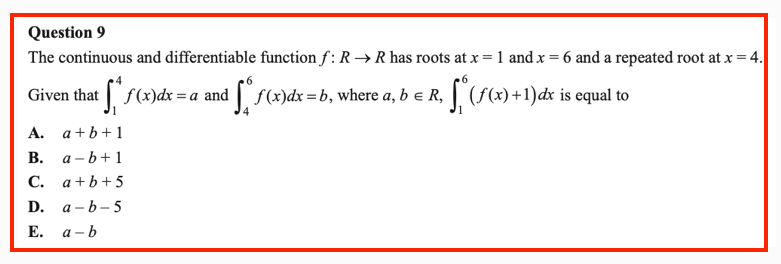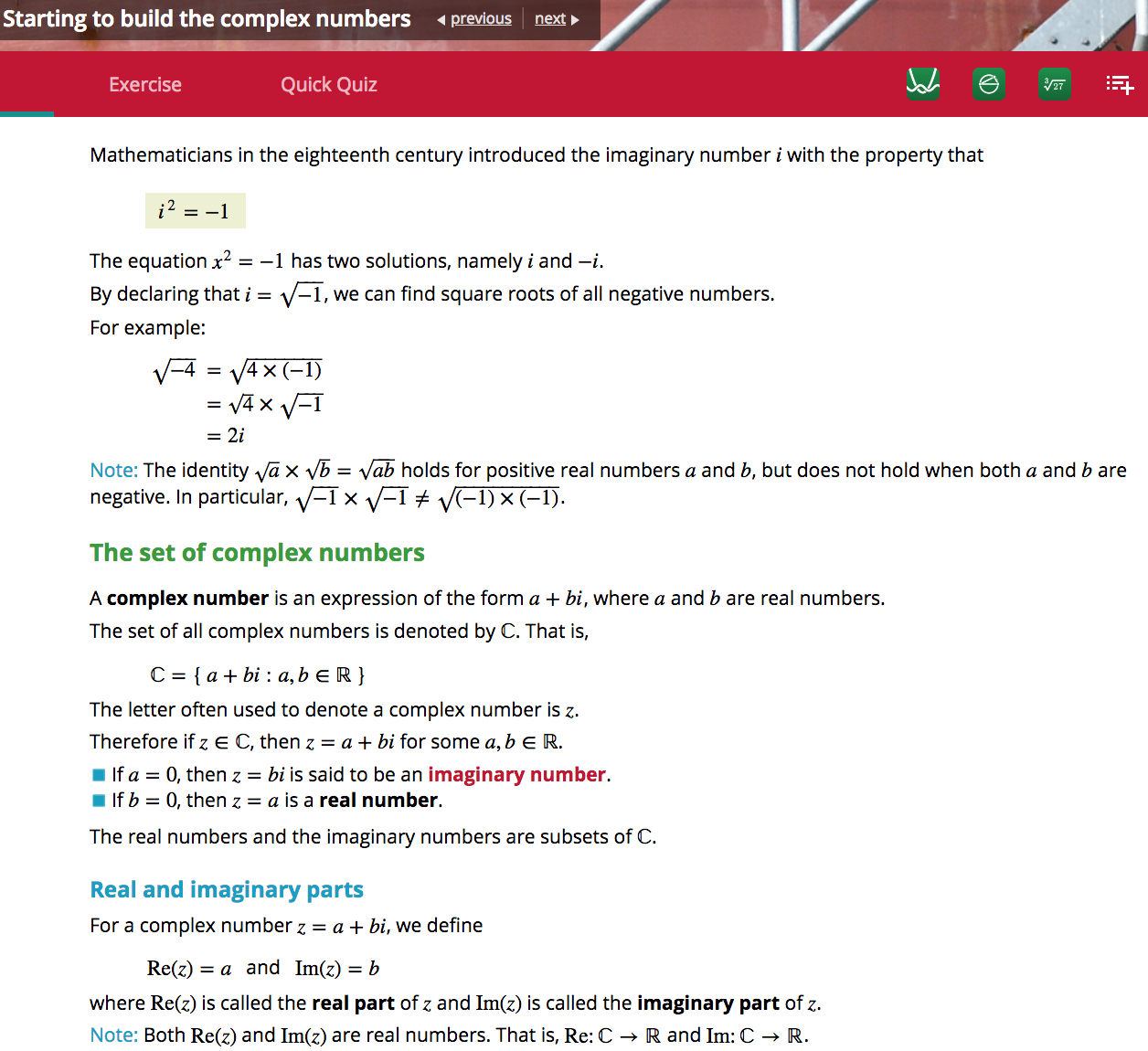## WitCH 65: Repeat Offender

The following is our second WitCH from the 2021 NHT Methods Exam 2.## MitPY 13: Trigonometry and Wolfram Alpha

This MitPY comes from frequent commenter, John Friend:

Dear Colleagues,

I gave a CAS-FREE question to my Specialist students whose first part was to solve (exactly) the equation. I solved it two different ways and got two different answers that are equivalent. I’ve attached my calculations.

I checked my answers using Mathematica, which lead to my question: Mathematica gives a third different but equivalent answer (scroll down to real solutions). How has Mathematica got this answer?

It may be that Mathematica ‘used’ my Method 2, got my tan answer and then for some arcane reason ‘manipulated’ this answer into the one it finally gives. If so, I can ascribe the answer to a Mathematica quirk. But it may be that Mathematica is using a method unclear to me that leads to its answer. If so, I’m curious.

Any thoughts are appreciated.

## MitPY 11: Asymptotes and Wolfram Alpha

This MitPY comes from frequent commenter, John Friend:

Dear colleagues,

I figured this was as good place as any to ask for help. I’m writing a small test on rational functions. One of my questions asks students to consider the functionwhereand to find the values offor which the function intersects its oblique asymptote.

The oblique asymptote isso they must first solve… (1)

for. The solution isand there are no restrictions along the way to getting this solution that I can see. So obviously.

It can also be seen that ifthen equation (1) becomeswhich has no solution. So obviously.

When I solve equation (1) using Wolfram Alpha the result is also. But here’s where I’m puzzled:

Wolfram Alpha gives the obvious restrictionbut also the restriction.emerges naturally (and uniquely) from this second restriction and I really like that this happens as a natural part of the solution process. BUT ….

I cannot see where this second restriction comes from in the process of solving equation (1)! Can anyone see what I cannot?

Thanks.

## MitPY 10: Square Roots

This MitPY comes from a student, Jay:

I have a question relating to polynomial equations. For context I have just finished Y11 during which I completed Further 3&4, Methods 1&2 and Specialist 1&2.

This year during my maths methods class we covered the square root graph, however I was confused as to why it only showed the positive solutions. When I asked about it I was told it was because the radical symbol meant only the positive solution.

However since then I have learnt that the graph ofalso only shows the positive solution of the square root, whileshows both. I am quite confused by why they aren’t the same. The only reason that I could think of is that it would meanwould be the same as, and while the points (-2,-4) and (2,-4) fit the latter they clearly don’t fit former.

Could you please explain why these aren’t the same?

## WitCH 2

Well, WitCH 1 is still not satisfactorily resolved, and Tweel’s puzzle is also still out there. But, we may as well get another ball rolling.

The second in our What is this Crap Here series comes from Cambridge’s textbook Specialist Mathematics VCE Units 3 & 4 (2018). Enjoy, and please get to pondering, and posting.## Update

Thanks to Damo for their hard work below.

The main problem with the above excerpt is that it should not exist. It is pointless to introduce complex numbers with more than a sentence on complex roots, and it is almost impossible to do so in a sensible manner. The nonsense of the text’s approach is encapsulated by the equationThis equation is best thought as false and, in the context of the excerpt above, must be thought of as meaningless. As is, thus, the discussion leading up to this equation.

How did they get there? To begin, i is introduced as a number for which i2 = -1, which is fine and good at the school level. Then, they note that the equation x2 = -1 has the two solutions i and -i, which is significantly less fine; since general complex numbers, and -i in particular, have not yet been defined, the notation -i is thus far meaningless, as is the notion of squaring this number. Still, if the sentence were more carefully worded, it would be reasonable in an introductory paragraph. The cavalier attitude to definition and meaning, however, is the sign of much worse to come.

The text continues by “declaring” that √(-1) = i, and then heads on its merry calculating way. But the calculation is complete fantasy. The declaration amounts to a (bad) definition of a specific root which cannot, in and of itself, tell us what any other root means or how it might be manipulated. So, √(-4) is as yet undefined, and the manipulation of this quantity is unjustified, as yet unjustifiable, and is best thought of as wrong.

In the real context we use √x to distinguish the positive root but it is fundamental that complex roots are multiple-valued. And, for the polynomial focus of VCE mathematics, multiple values are perfectly fine and perfectly natural. The quadratic formula remains true without change and the purportedly troublesome identityis always true (modulo the understanding that if x is a positive real then√x is now ambiguous). Moreover, with this natural interpretation, the text’s declaration that √(-1) = i is false, as is the equation √(-4) = 2i.

Admittedly, at some point it is valuable, and essential, to introduce principal values of roots, by which the text’s equation can be interpreted to be true. But principle roots are intrinsically awkward, must be introduced with great care and should only be introduced when there is a purpose. Which is not on page 1 of a school text, and arguably not ever in a school text.

Apart form the utter pointlessness and utter meaningless of the excerpt, we note:

• The text conflates the introduction of imaginary numbers in the 16th century with the introduction of the symbol i in the 18th century.
• The text implies 0 is an imaginary number, which is ok though a little peculiar.
• The real numbers and imaginary numbers are not subsets of.
• The characterisationsandare grandiose and pointless.